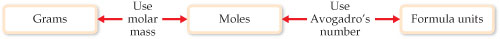# Problem: Procedure for interconverting mass and number of formula units. The number of moles of the substance is central to the calculation. Thus, the mole concept can be thought of as the bridge between the mass of a sample in grams and the number of formula units contained in the sample.What number would you use to convert moles of CH4 to grams of CH4?

###### FREE Expert Solution

For methane, CH4

1 mol CH4 → molar mass CH4 → mass CH4

96% (119 ratings)###### Problem DetailsProcedure for interconverting mass and number of formula units. The number of moles of the substance is central to the calculation. Thus, the mole concept can be thought of as the bridge between the mass of a sample in grams and the number of formula units contained in the sample.

What number would you use to convert moles of CH4 to grams of CH4?

Frequently Asked Questions

What scientific concept do you need to know in order to solve this problem?

Our tutors have indicated that to solve this problem you will need to apply the Mole Concept concept. You can view video lessons to learn Mole Concept. Or if you need more Mole Concept practice, you can also practice Mole Concept practice problems.